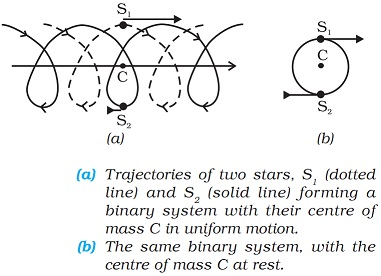# System of Particles and Rotational Motion - Online Test

Q1. Rigid body is a body which
Explaination / Solution:

A rigid body is an idealization of a solid body in which deformation or change in shape is neglected on application of deforming force.

Q2. In pure translational motion of a rigid body
Explaination / Solution:

In translational motion when the body moves along a straight line or more exactly when every point of the body travels on paralell lines, thus at any instant of time every particle of the body has the same velocity.

Q3. In rotation of a rigid body about a fixed axis is that in which
Explaination / Solution:

When a rigid body rotates about a fixed axis, all particles of the body except those which lies on the axis of rotation, move along circular paths in a plane perpendicular to the axis.

Q4. In precession such as that of a top
Explaination / Solution:

As precession is a change in the orientation of the rotational axis of a rotating body, so the orientation of axis of rotation of Top change

Q5. In a general motion of a rigid body
Explaination / Solution:

In general motion of the body can be seen as the combination of pure translation of the center of mass and pure rotation of the body about the center of mass like the motion of a wheel.

Q6. the centre of mass of a system of particles moves
Explaination / Solution:

The centre of mass of a system of particles moves as if all the mass of the system is concentrated at the centre and all the external forces were applied at that point. In order to ease the calculations involved in the dynamics of an extended body, we treat any extended body as the system of particles, and the motion of centre of mass of the system is calculated by taking the mass of the whole system to be concentrated at the centre of mass and the external forces acting on system are said to be acting on the centre of mass.

Q7. considering binary (double) stars in our frame of reference, the trajectories of the stars are a combination of
Explaination / Solution:

A double star or visual double is a pair of stars that appear close to each other in the sky as seen from Earth when viewed through an optical telescope.

In absence of  external force Centre of mass of double star moves like a free particle. In Centre of mass frame both stars moving in a circle about the Centre of mass which is at rest and both star are diametrically opposite to each other.

Thus in our frame of reference, the trajectories of the stars are a combination of (i) uniform motion in a straight line of the Centre of mass and        (ii) circular orbits of the stars about the Centre of mass.Q8. The vector product of two vectors a and b is a vector c such that the magnitude of c is given by
Explaination / Solution:

As per definition of vector product :-

Q9. The vector product of two vectors a and b is a vector c such c is perpendicular to the plane containing a and b and the direction is given by is given by
Explaination / Solution:

If vectors A and B lie in the plane of this page, the vector C will be perpendicular to this plane.
The sense (upward or downward) of the direction of the vector product is given by the direction of the advance of the tip of a right-handed screw when rotated from A to B through angle  between them, the screw being placed with its axis perpendicular to the plane containing the two vectors.

Q10. If a body is rotating about z axis with a speed ω and a point is at a distance of r in the x-y plane then the velocity of the point is

Explaination / Solution:

angular displacement of particle about z axis

differentiate with respect to time